# ISEE Upper Level Quantitative : How to find a ray

## Example Questions

### Example Question #1 : How To Find A Ray

Which is the greater quantity?

(a) The length of the line segment connecting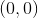and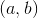(b) The length of the line segment connecting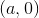and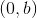(a) and (b) are equal.

It is impossible to tell from the information given.

(b) is greater.

(a) is greater.

(a) and (b) are equal.

Explanation:

(a) The length of the line segment connectingandis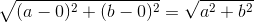.

(b) The length of the line segment connectingandis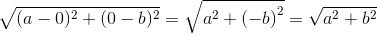.

The segments have equal length.

### All ISEE Upper Level Quantitative Resources